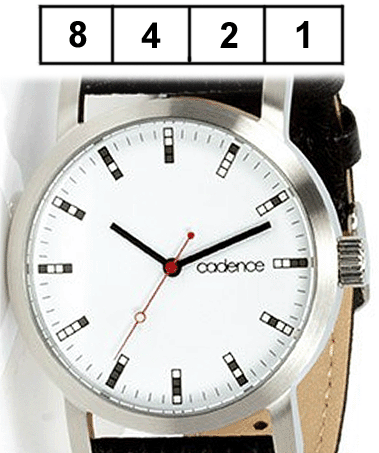# Definition: binary

(1) A binary is an executable program. See bin and bin file.

(2) Meaning two. Binary is the principle behind digital computers. All input to the computer is converted into binary digits that are either 0 or 1. For example, when you press the "A" key on your keyboard, the keyboard circuit generates and transfers the number 01000001 to the computer's RAM as a series of pulses with different voltages. The bits are stored as temporarily charged cells in RAM, as permanent charges in a solid state drive (SSD) or as microscopic magnets on a hard drive. The computer's display screen and printers convert the binary numbers into visual characters.

Circuits Are Binary
The electronic circuits that process these binary numbers are also based on binary. They are made up of on/off switches (transistors) that are electrically opened and closed. The current flowing through one switch turns on (or off) another switch, and so on. These switches open and close in nanoseconds and picoseconds (billionths and trillionths of a second). See Boolean gates.

Smaller Spots - Faster Switches
A computer's capability to do work is based on its workspace capacity (RAM), storage capacity (disk or SSD) and the speed of its circuits. Greater capacities are achieved by making the memory cells or magnetic spots smaller. Faster circuit speeds are achieved by shortening the time it takes to open and close the transistor (electronic switch). In order to increase computer performance, we keep improving binary technologies. See binary numbers, binary values, binary file, binary standard and binaries.

How Binary Numbers Work

In the decimal numbering system, adding 9 and 1 produces a result of 0 in the 1s position plus a carry of 1. The carry jumps over to the 10s position on the left.

```             carry--1
9
+  1
____
10
```

Binary numbers use only the digits 0 and 1. The following example adds 1 ten times in succession in both base 2 (binary) and base 10 (decimal). The carry occurs many more times in binary because there are only two digits (0 or 1).

```              Binary      Decimal

0           0

+  1        +  1
____        ____
1           1

+  1        +  1
____        ____
10           2

+  1        +  1
____        ____
11           3

+  1        +  1
____        ____
100           4

+  1        +  1
____        ____
101           5

+  1        +  1
____        ____
110           6

+  1        +  1
____        ____
111           7

+  1        +  1
____        ____
1000           8

+  1        +  1
____        ____
1001           9

+  1        +  1
____        ____
1010          10
```For the True Geek
This analog wristwatch displays the hours in binary. Only four bits (1, 2, 4 and 8) are required to hold 12 hours. The hour hand is currently at 10 o'clock, which shows the 2-bit and 8-bit turned on. (Image courtesy of Cadence Watch Company.)